# gpu 0: Software rasterizer, part 1

2020-10-17

I would like to design some hardware to accelerate raster graphics. However, I need to first have a better understanding of the subject. A good method for this may be to implement some of the graphics pipeline in software and put myself in the shoes of a graphics programmer in the 80s.

Let's jump into the middle of the pipeline and write a triangle rasterizer, which is a machine that turns triangles into pixels. From there we can expand out to both directions as needed.

At the beginning, I will follow Triangle rasterization in practice from ryg which explains the process very well. So, if this post comes across like a walkthrough of ryg's post, you know why.

I'll implement the rasterizer in JS and use the canvas element to draw. This way I can write it together with the blog post and have a "live code" environment.

A javascript canvas.

Here we have some place to draw with chunky pixels and a grid to make error spotting easier. It's a 32x32 canvas scaled up by a factor of 4. I start with a triangle on canvas space coords (8, 28), (15, 3) and (25,10).

A javascript canvas.

## 2D rasterizer

After looking at The barycentric conspiracy for a little too long, I got that the basic technique is to calculate signed areas of every triangle formed by one edge of our original triangle and our point. If all are positive, the point lies to the left of all edges and should be painted. For the v10 edge and the point p, the signed area is calculated as: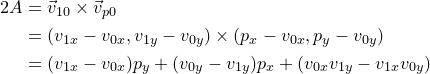If p is to the left of v10, the vp0 vector lies to the left of v10 and the cross product should be positive from the right hand rule.

Another thing to note is that the edge function which yields this signed area is affine, so you can just add (v1x - v0x) to the value calculated with py and get the value for (py + 1).

The other two edge functions are: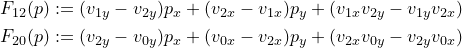Let's implement this in a bunch of JS.

function edge_fn(v0, v1, p){
return (v0.y - v1.y)*p.x + (v1.x - v0.x)*p.y + (v0.x*v1.y - v1.x*v0.y);
}

function draw_trig(v0, v1, v2){
/* triangle bounding box */
var minX = Math.min(v0.x, v1.x, v2.x);
var minY = Math.min(v0.y, v1.y, v2.y);
var maxX = Math.max(v0.x, v1.x, v2.x);
var maxY = Math.max(v0.y, v1.y, v2.y);

/* clip to screen coords */
minX = Math.max(minX, 0);
minY = Math.max(minY, 0);
maxX = Math.min(maxX, W-1);
maxY = Math.min(maxY, H-1);

ctx2.fillStyle="#f00";
for(var y=minY; y<=maxY; y++){
for(var x=minX; x<=maxX; x++){
p = {x: x, y: y};
if(edge_fn(v0, v1, p) >= 0 &&
edge_fn(v1, v2, p) >= 0 &&
edge_fn(v2, v0, p) >= 0){
/* put pixel */
ctx2.fillRect(x, y, 1, 1);
}
}
}
}


Note that we only traverse the bounding box of the triangle, not the whole screen. The output is, as expected, a triangle:

A javascript canvas.

Now, there is nothing wrong with this piece of code except that it's slow and incorrect. It computes the whole edge function for every pixel, samples from the wrong point of the pixel and does not apply any fill rules.

Let's add a bigger pixel grid with marked pixel centers like this guide and check what's wrong with our basic approach. By the way, I didn't know the Microsoft programming guide for D3D11 included a full description of the graphics pipeline with nice illustrations. A new addition to the reading list.

Here are the lit-up pixels with our approach:

A javascript canvas.

You can see that it's quite off since we are sampling from the top left corner of every pixel.

I think 1 bit of sub-pixel precision is enough to sample pixels from the middle point. However, we can go all the way and put 8 bits of sub-pixel precision as required by DX11. That requires us to multiply everything with 256 and round to the nearest integer. This way we can emulate X.8 fixed point arithmetic using JS numbers.

function draw_trig(v0, v1, v2){
/* clamp to nearest subpixel */
var x0 = Math.round(v0.x * 256);
var y0 = Math.round(v0.y * 256);
var x1 = Math.round(v1.x * 256);
var y1 = Math.round(v1.y * 256);
var x2 = Math.round(v2.x * 256);
var y2 = Math.round(v2.y * 256);

/* triangle bounding box */
var minX = Math.min(x0, x1, x2);
var minY = Math.min(y0, y1, y2);
var maxX = Math.max(x0, x1, x2);
var maxY = Math.max(y0, y1, y2);

/* clip to screen coords */
minX = Math.max(minX, 0);
minY = Math.max(minY, 0);
maxX = Math.min(maxX, (W-1)<<8);
maxY = Math.min(maxY, (H-1)<<8);

/* replace fractional part with 128 to sample from center */
minX = (minX & ~255) + 128;
minY = (minY & ~255) + 128;
maxX = (maxX & ~255) + 128;
maxY = (maxY & ~255) + 128;

for(var y=minY; y<=maxY; y+=256){
for(var x=minX; x<=maxX; x+=256){
if(edge_fn(x0, y0, x1, y1, x, y) >= 0 &&
edge_fn(x1, y1, x2, y2, x, y) >= 0 &&
edge_fn(x2, y2, x0, y0, x, y) >= 0){
/* remove fractional part before putting pixel */
ctx4.fillStyle="rgba(255, 0, 0, 0.3)";
ctx4.fillRect((x >> 8) * 16, (y >> 8) * 16, 16, 16);
}
}
}
}


This looks much better:

A javascript canvas.

Next, fill rules. To demonstrate, I'll add a shape from the D3D10 guide. Our rasterizer currently draws the shape like this:

A javascript canvas.

As you can see, the middle 3 pixels are colored twice. That is what fill rules should be preventing. They come into work when the edge of a triangle crosses the middle of a pixel, i.e. the edge function is 0 at that point:

Any pixel center which falls inside a triangle is drawn; a pixel is assumed to be inside if it passes the top-left rule. The top-left rule is that a pixel center is defined to lie inside of a triangle if it lies on the top edge or the left edge of a triangle.

Where:

• A top edge, is an edge that is exactly horizontal and is above the other edges.
• A left edge, is an edge that is not exactly horizontal and is on the left side of the triangle. A triangle can have one or two left edges.

This looks confusing, but they get easier if we consider triangles wound in only one direction. For clockwise triangles (as in canvas coords):

• A top edge is an edge that is exactly horizontal and goes to the right. (Otherwise we get a CCW triangle.)
• A left edge is an edge that goes up. Since it goes up, it can't be exactly horizontal.

Let's go ahead and write the code. We'll add a bias value of -1 for non top-left edges. That will prevent drawing of the pixel in case the edge function ends up at 0.

function is_top_left(x0, y0, x1, y1){
if(y0 == y1 && x0 < x1) return true;
else if(y0 > y1) return true;
else return false;
}

function draw_trig(v0, v1, v2, color){
/* ... */

/* fill rules */
var bias0 = is_top_left(x1, y1, x2, y2) ? 0 : -1;
var bias1 = is_top_left(x2, y2, x0, y0) ? 0 : -1;
var bias2 = is_top_left(x0, y0, x1, y1) ? 0 : -1;

for(var y=minY; y<=maxY; y+=256){
for(var x=minX; x<=maxX; x+=256){
var w0 = edge_fn(x1, y1, x2, y2, x, y) + bias0;
var w1 = edge_fn(x2, y2, x0, y0, x, y) + bias1;
var w2 = edge_fn(x0, y0, x1, y1, x, y) + bias2;
if(w0 >= 0 && w1 >= 0 && w2 >= 0){
/* remove fractional part before putting pixel */
ctx6.fillStyle = color;
ctx6.fillRect((x >> 8) * 16, (y >> 8) * 16, 16, 16);
}
}
}
/* ... */
}


Now the result should be identical to the one in the D3D10 guide. Actually, let's add a few more shapes from the guide to check if they get rasterized correctly:

A javascript canvas.

Looks OK. After we get here, ryg goes on to optimize this rasterizer, but I think we should first get to perspective projection. If we complete that part of the pipeline, we can draw more complex 3D scenes which will eventually require a fast rasterizer.

## Perspective projection

In my opinion, the best part of raster graphics is that you can just paste a 3D scene onto the screen and work there with a series of linear interpolations. At least, this is my view as someone who only knows about ray tracing.

(I will be following chapter 18 of this 1996 book, this post from Simon and this WebGL guide for this section.)

On a closer look, it seems like that small pasting 3D to 2D step is a little complicated. We have to first convert to a clip space, do clipping and then convert to viewport coordinates. Furthermore, the transform is done in 4D projective space, something I have never had to think about in ray tracing. In ray tracing everything is 3D and the scene clips itself...

I had a hard time wrapping my head around how we embed the properties of our viewing frustum into the perspective transform matrix. How do we move the camera? Where is the near plane? Far plane? The mapping to clip space? It took some staring at the resources to get a hold of the situation. To quote the steps of the transformation from the WebGL guide:

We need to perform the following steps to create a perspective projection transformation matrix:

1. Translate the apex of the frustum to the origin.
2. Perform the perspective calculation.
3. Scale the 2D (x’,y’) values in the viewing window to a 2-by-2 unit square: (-1,-1) to (+1,+1).
4. Scale the depth values (z) into a normalized range (-1,+1).
5. Flip the orientation of the z axis to match the clipping volume’s orientation.

The tricky steps here are 2 and 4. Step 2 is easier than it looks, it's the old triangle similarity math which turns X into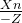. Here,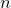is the near plane distance and Z is negative since the camera is assumed to be looking down the -Z direction.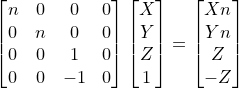Step 4 should map Z to 0 (for DirectX, -1 for OpenGL) at the near plane and 1 at the far plane instead of locking it to -1. That requires some tuning of the matrix parameters.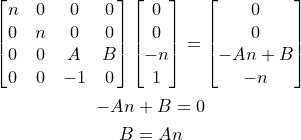Then we map the far plane z = -f to z = 1: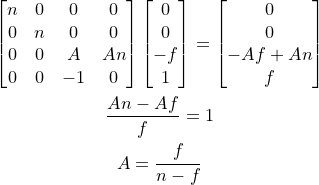Let's finally apply the viewport mapping to (-1, +1) and assume the camera has no XY offset. This brings us to our final perspective transform matrix: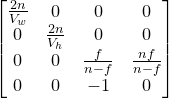This matrix takes us to the clip space. After clipping we normalize by w and then map X and Y to the viewport coordinates. The resulting screen space is probably where our rasterizer should work. (Not the canvas coords!)

For the first test, I am going to use the camera and vertex data from the scene in rt 1. The scene only has some shapes on the Z=-2 plane, so it shouldn't require clipping or depth buffering. (Ignoring the sphere for now.)

var cam = {vw: 2, vh: 2, near: 1, far: 100};

function proj_mtx(vw, vh, n, f){
return [
[2*n/vw, 0, 0, 0],
[0, 2*n/vh, 0, 0],
[0, 0, f/(n-f), n*f/(n-f)],
[0, 0, -1, 0]
];
}

function viewport(v, w, h){
return [(v+1)*w/2, (v+1)*h/2, v, v];
}

function wnorm(v){
return [v/v, v/v, v/v, 1];
}

var P = proj_mtx(cam.vw, cam.vh, cam.near, cam.far);

var verts_vp = [];
for(var i=0; i<verts.length; i++){
var v = verts[i];
var v_clip = mat4x4_mul_vec4(P, v);
var v_vp = viewport(wnorm(v_clip), 128, 128);
verts_vp.push(v_vp);
}

/* draw triangles */
...


A javascript canvas.

Looks like our matrix worked: vertices are at the right coords. Here is the ray tracer's rendering of the scene for comparison.

Let's get back to the rasterizer now. I think this is a good time to start using viewport coordinates instead of canvas coordinates. That should only reverse the left edge rule:

function is_top_left(x0, y0, x1, y1){
if(y0 == y1 && x0 < x1) return true;
else if(y0 < y1) return true;
else return false;
}


And, here is the result:

A javascript canvas.

I think this is officially the longest path to drawing a square and a triangle on an HTML canvas :) Anyway, we have still a long way to go in the pipeline: we still don't have clipping, depth buffering, textures etc., and our rasterizer is not optimized. We will deal with those in the next post. See you until then!